Speed devil of an RNA engine

• Idea
• Updated 1 year ago
• (Edited)

Speed devil of an RNA engine

I have through my eterna time seen a bunch of different RNA algorithms and I have in general not been too impressed. I usually submit them to a battery of tests, like throw them lab designs that we have the structure for, to see if they can correctly predict the structure. Usually they come out short on the prediction side.

Omei introduced me to a new RNA folding algorithm yesterday. Hereby comes my chat with him on the new folding engine.

Eli: Loose thought, the wuami charts seems faster with the calculation. I was wondering if they could somehow be used. Like instead of a melt plot. As a guiding feature while working a puzzle

Omei: Speaking of which: LinearFold: Linear-Time Prediction of RNA Secondary Structures https://www.biorxiv.org/content/early/2018/02/14/263509

bioRxiv

LinearFold: Linear-Time Prediction of RNA Secondary Structures

Predicting the secondary structure of an RNA sequence with speed and accuracy is useful in many applications such as drug design. The state-of-the-art predictors have a fundamental limitation: they have a run time that scales with the third power of the length of the input sequence, which is slow for longer RNAs and limits the use of secondary structure prediction in genome-wide applications. To address this bottleneck, we designed the first linear-time algorithm for RNA secondary structure prediction, which can be used with both thermodynamic and machine-learned scoring functions. Our algorithm, like previous work, is based on dynamic programming (DP), but with two crucial differences: (a)... Show more

Feb 14th

Eli: this sounds interesting. So what I hear you thinking is that perhaps there is a solve to our problem [Long lab calculation time], elsewhere. This is kind of like you moving our puzzle to log concentrations instead of multiplication

Omei: Yes, I've also considered how to incorporate the wuami charts into the design process and came to the same thought -- replacing the melt chart.  It's an easy incremental change.

Omei: "I think the wuami charts require essentially the same computation as the current state 4 ones.", i.e. take about the same time.

Eli: I think it is way more useful than any energy calculation of the model. I work in frozen mode a lot of the time anyway to avoid having to wait for calculation

Eli: It would even show pretty chart for promising unstable designs.

LinearFold Webserver

Eli: Ha, I found a web server for LinearFold

http://linearfold.eecs.oregonstate.edu:8080/

And I forgot to mention, there is no wait time for the server. Although the puzzle I feed it is around 3-4 times longer than any of our labs.

Eli: Feed it one of my monsters.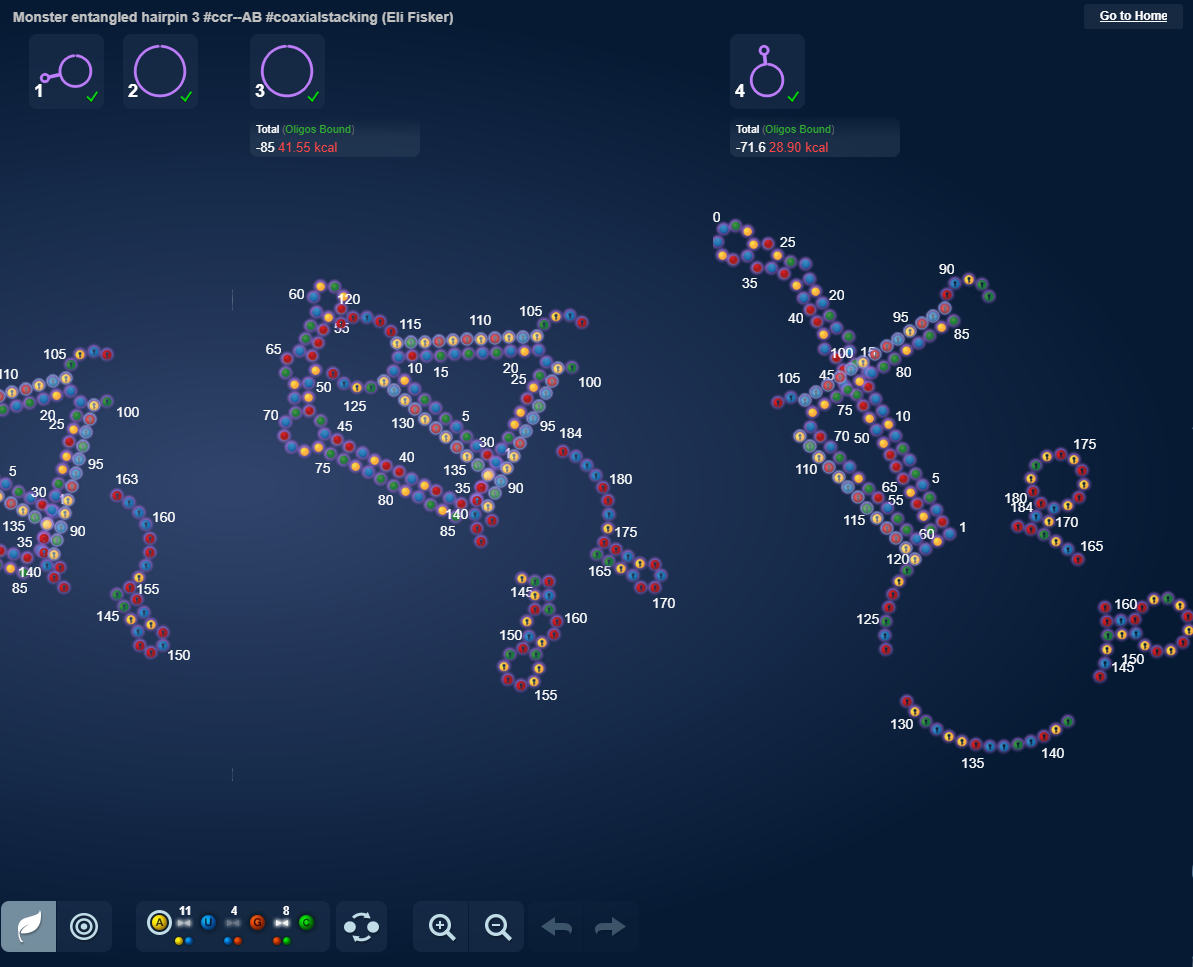UGUCUCUCUAUUGUCUCUCUAUUCAGAACUUAGUGUGAUGGAUGGUCGAUAGGGAGACAUUCCUGCAUCUGUAACCAUCCAUCAC

http://www.eternagame.org/game/browse/8489874/?filter1=Id&filter1_arg2=8709857&filter1_arg1=8709857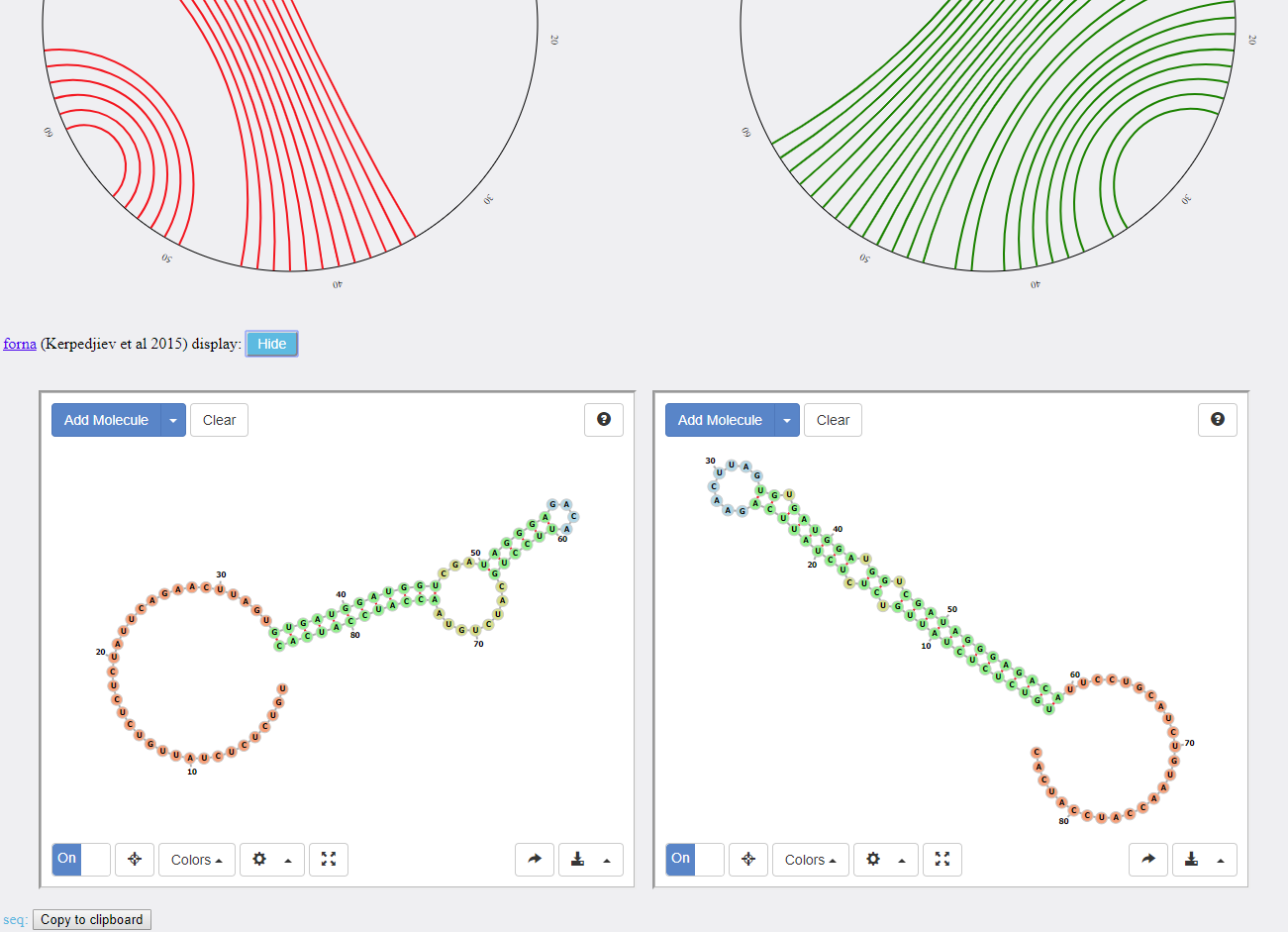Eli: Shows both the structures we want forming. As dangle bases without the inputs

Eli: This time I made a switch puzzle a different way. I simply nicked one of the dot bracket structures that linear fold spit out from my entering my puzzle sequence through the server. It made a rather pretty alternative structure. Then I solved the puzzle like I usually would have. Okay I swapped the puzzle to Vienna2, as the new structure would easier get stable there.

Eli: Now I got it thinking. I threw it a sequence of a bigger snowflake puzzle. :) But I did get output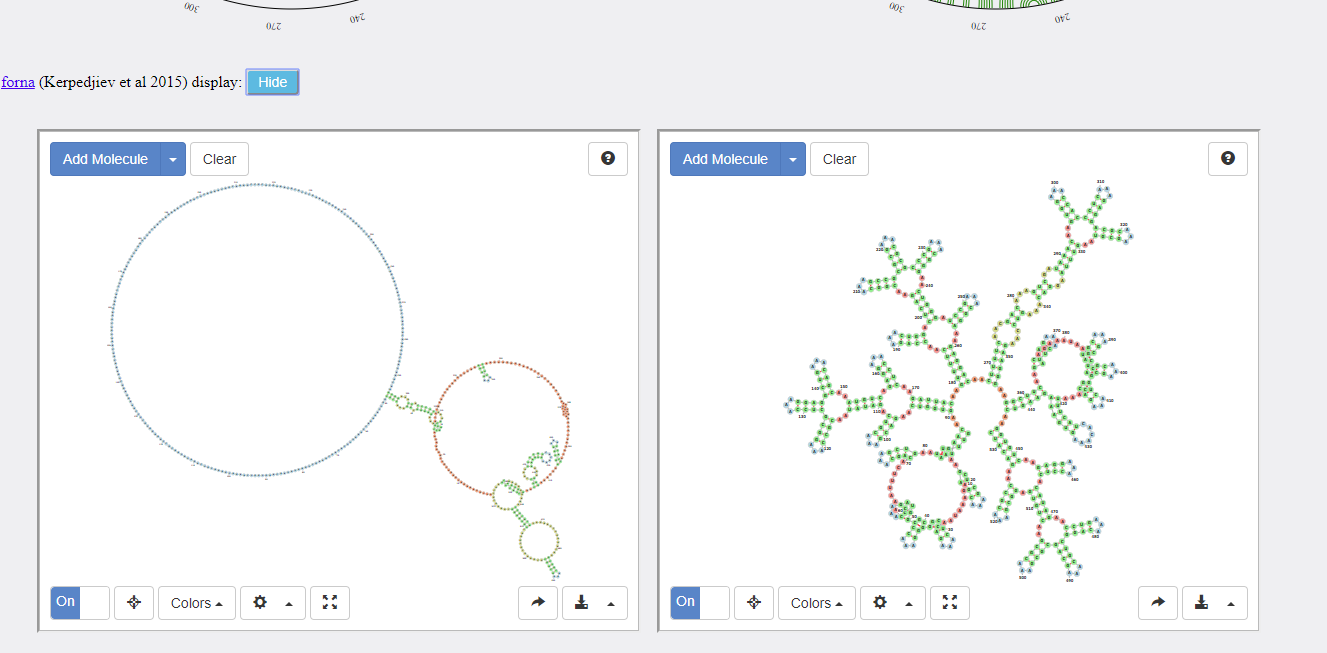Eli: I rather like what it did. It predicts a bunch of misfolds. (Which is rather realistic.)

I gave it my sequence for this puzzle:

http://www.eternagame.org/game/puzzle/13833/

I'm also consider throwing it a bunch of past lab designs that we have data on how should fold.

Eli: It is not doing bad on the latter. It has just predicted the structure we expect of a big hairpin design. The other I tested was the top scorer of Cloud Lab 13 - Alien Party Glasses by Jennifer Pearl. So far I'm rather impressed. I don't recall any other RNA web server getting this close on actual design structures we have had in lab.

Eli: I am super impressed. I can almost use it to recreate the structure of one of my switch puzzles.

Here is my original shape:http://www.eternagame.org/game/puzzle/8568428/

I throw it my solve.

If I open the puzzlemaker for two shapes and plot in both the structure that both linearFold V and C outputs then I get an almost stable puzzle back

LinearFold-C: Copy to clipboard

..((((((((....))))..((((((((((((((..((((....))))((((....))))((((....))))..))))))))))))))..((((....((((((((....))))((((....))))..((((((((((((((..((((....))))((((....))))((((....))))..))))))))))))))..))))....))))))))..

LinearFold-V: Copy to clipboard

(.((((((((....))))..((((((((((((((..((((....))))((((....))))((((....))))..))))))))))))))..((((....))))((((....))))((((....))))..((((((((((((((..((((....))))((((....))))((((....))))..))))))))))))))..((((....)))))))).)

I added in a molecule and only one base pair that are supposed to be fold, are not.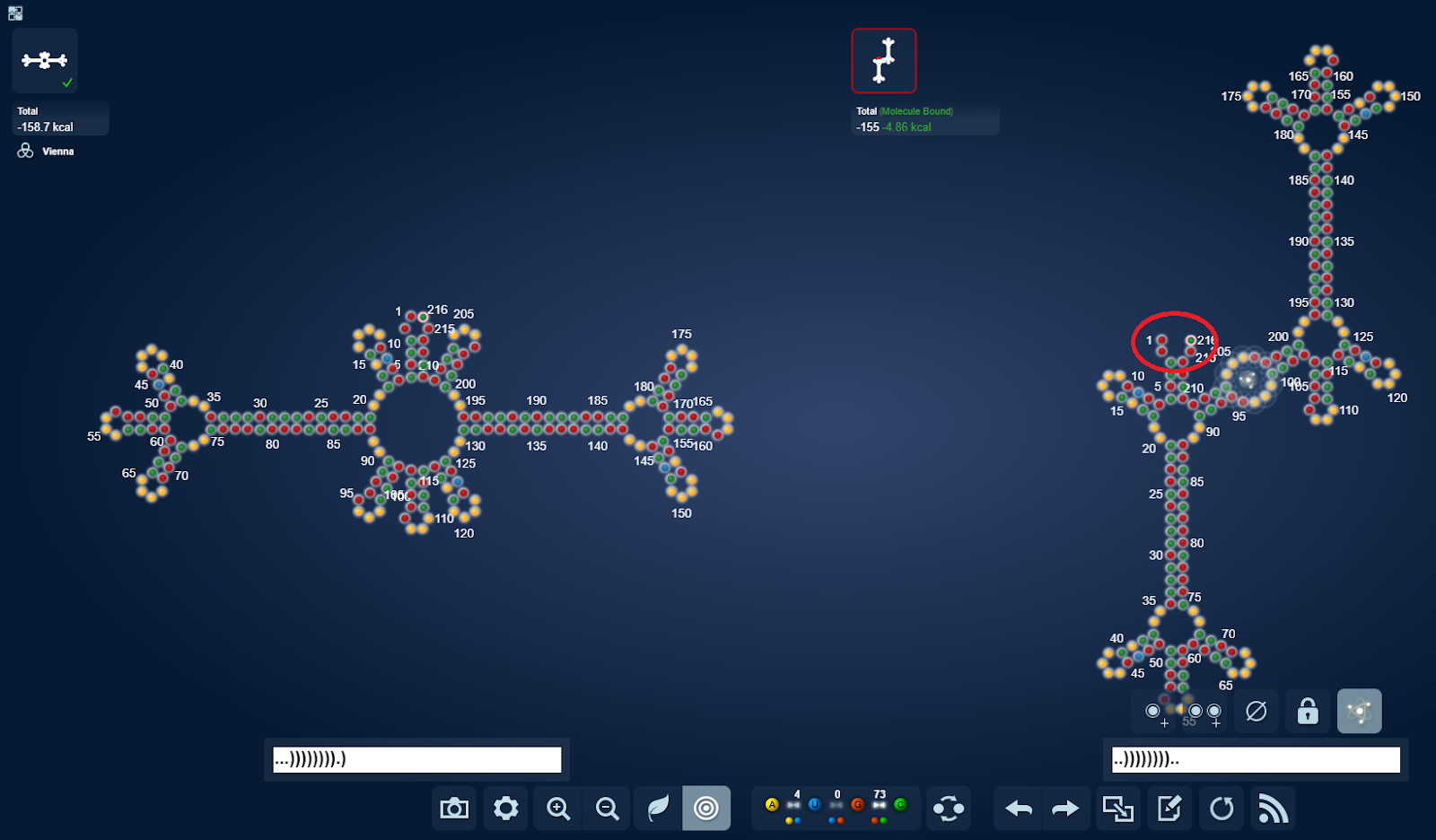Solving sequence:

GGCCGCGGUGAAAACACCAACCGGCGCCCGCCCGAACCACAAAAGUGGCCGGGAAACCGGGGCCAAAAGGCCAACGGGCGGGCGCCGGAACCGGGAAACCGGGCGGGAAACCGCGCACAAAAGUGCAACCGGCGCGGGCCGGAAGCUCAAAAGAGCGCCGGAAACGGCGGCCAAAAGGCCAACCGGCCCGCGCCGGAACCGGGAAACCGGGCGGGC

Omei: Interesting.  Are you observing that LinearFold-C tends to predict one state's folding while LinearFold-V predicts the other?

Eli: Yes. So far the Vienna one seems more accurate for the past single state labs. At least for what I have observed so far.

Omei: That, I would expect.

Eli: Also when I plot in the lab designs back into the puzzlemaker to view them in eterna, I sometimes have to swap to vienna2 to get them stable

Omei: If LinearFold-C tends toward finding the other state, that comes as a surprise.

Eli: And I ran the switch topscorer from Top notch

Omei: These are not specifically intended to separate the two states of our puzzles.

Eli: When I put it in nupack, the native fold and the target structure almost resembled the switch.

I know, I just couldn't help myself. :)

And I'm amazed with what I see

Basically I haven't seen anything this good before

Omei: Can you elaborate on "When I put it in nupack, the native fold and the target structure almost resembled the switch".

Eli: I took the sequence from Hotcreek's winner:

http://www.eternagame.org/game/solution/2426190/2449484/copyandview/

I put the sequence through LinearFold

I took the structure from LinearFold-V:

..........((((.....((((((..............)))))).(((((....)))))..))))..(((((((....))))))).....................

I put it in the single state puzzlemaker. And changed engine to nupack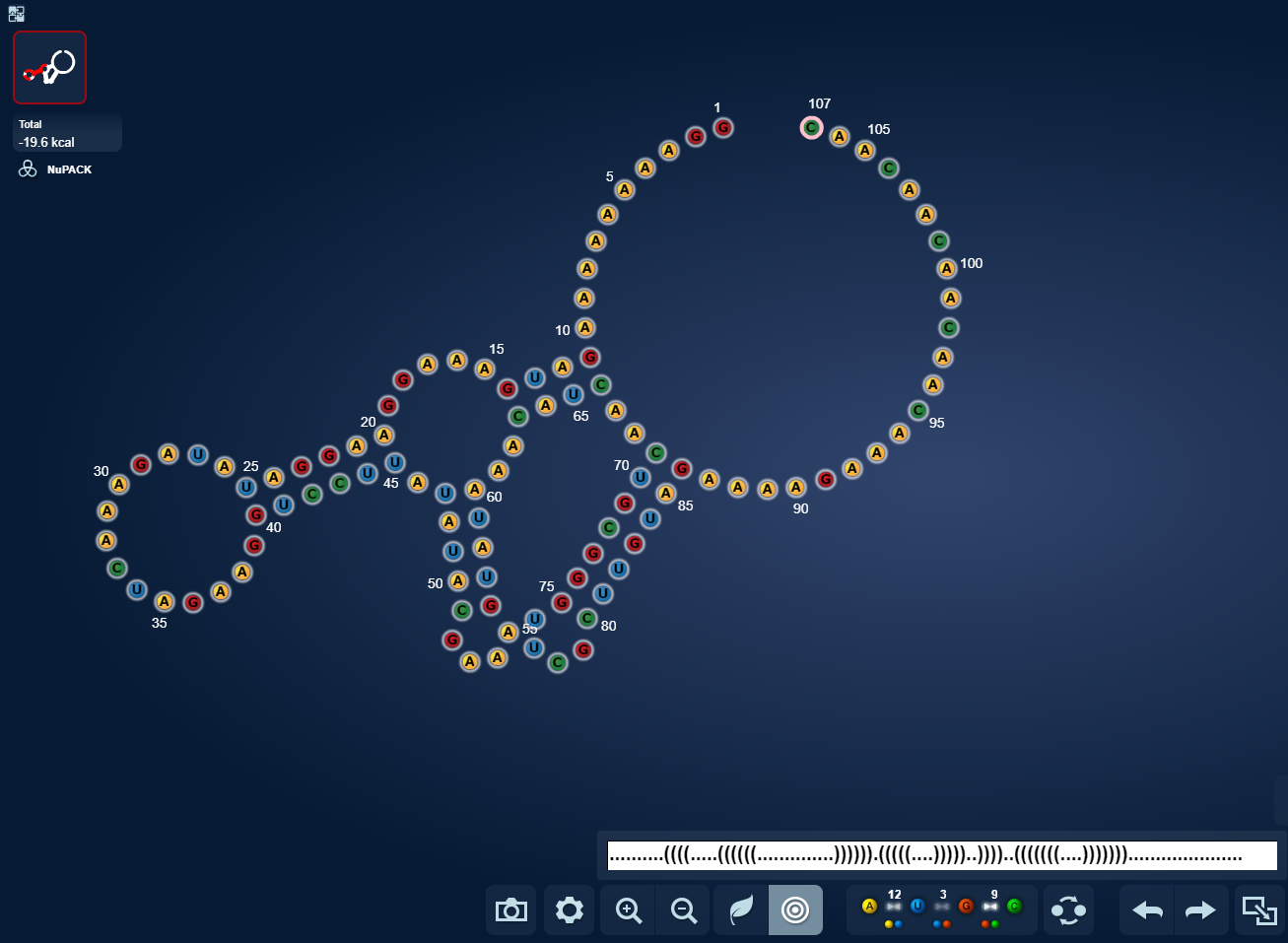http://www.eternagame.org/game/puzzlemaker/

Sequence:  GGAAAAAAAAGAUGAAAGGAAGGAUAUAGAAACUAGAAGGUCCUUAUAUACGAAAGUAUAAACAUCAACUGCGGGUUCGCUUGUAGAAAAGAAACAACAACAACAAC

When I swap back and forth between native and target, I get real close to what the actual switch look like

There is a small hairpin stem not forming in target state. But it is real close to what the real switch structure looks like

Omei: Hm. LinearFold-V uses the Vienna thermodynamics parameters.  So I would expect it to usually reproduce what we see in Vienna2.

Eli: Aha, that will explain why I regularly have to swap to Vienna2 to get a stable design.

But what I was fascinated with here was that I could use Nupack to show the switching and that LinearFold allowed me to get back part of the switch structure and the sequence in combo with Nupack would get me part of the rest.

LinearFold + Eterna?

Extra discussion from today:

Omei: Yes.  If I seem less enthusiastic than you, it is only because I don't understand yet what it gives up to achieve the linear time.  The web server doesn't give a partition function display, for example.

Omei: Since the web version, at least, doesn't have any facility to model small molecules or oligos, it's hard for me to see how we can judge "accuracy".

Eli: I think what it is really good at is seeing what parts of a puzzle are intended to be together. Actually regardless of weather they would form in lab or not.

Omei: Yes, that gets to what does seem promising to me.

If we can use it to create relevant real-time feedback while designing, that should be useful, even if is only a first approximation that needs additional vetting.

Eli: Could save a ton of calculations

Omei: I actually think for OpenTB puzzles, we could write write code that indicates what parts of a puzzle are intended to bind, without any thermodynamic calculations.